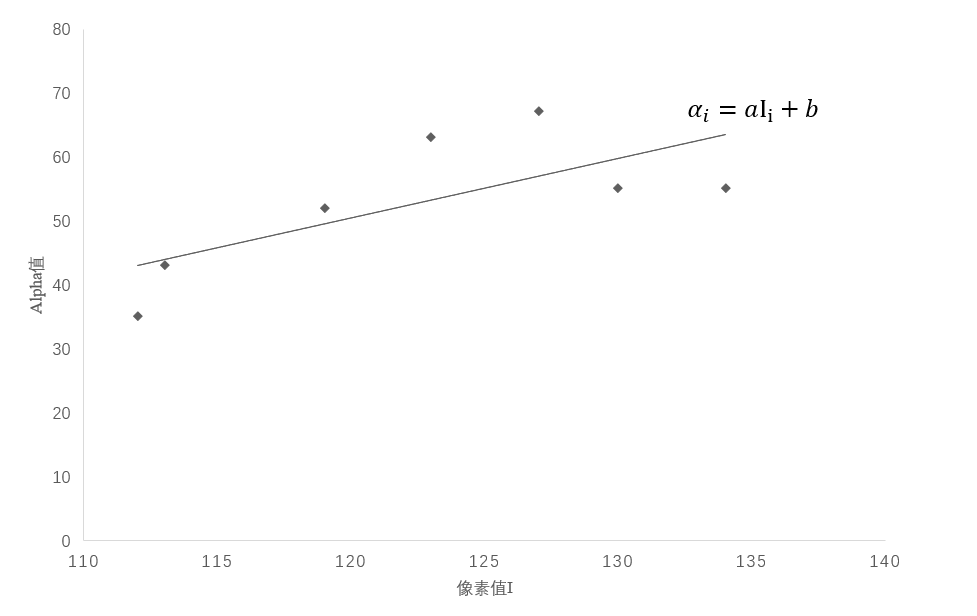# A Closed Form Solution to Natural Image Matting – 笔记

2020-7-17

### # 完整计算流程$G_k=\begin{bmatrix} I_i^k & 1\\ I_2^k & 1\\ \vdots & \vdots\\ I_w^k & 1\\ \sqrt{\epsilon} & 0 \end{bmatrix}$$\bar{\alpha_k}=\begin{bmatrix} \alpha_1^k\\ \alpha_2^k\\ \vdots\\ \alpha_w^k\\ 0 \end{bmatrix}$，则上式写成：

$\left \| AX-B \right \|^2$的梯度，令其梯度为0得最优解：

$\bar{G_k}=I-G_k(G_k^TG_k)^{-1}G_k^T$$I$代表单位矩阵），则：

$J(\alpha)$写成：

$\bar{G_k^T}\bar{G_k}$展开可得$L$的第$i,j$项（文末推导$L$

（其中$S$为包含scribbles的图像数据，是一个$N\times1$的矩阵。 $D_c$用于指示scribbles覆盖的像素的位置，是一个$N\times N$的对角矩阵， 主对角线上的$N$个值，属于scribbles的位置为1，矩阵其余位置都为0。）

### # 推导$L$的第$i,j$项

$G_k^TG_k$可写成：

$G_k^TG_k$的逆：

$\frac{1}{w\sigma^2+\epsilon}$$k_1$ $\sigma^2+\mu^2+\frac{\epsilon}{w}$$k_2$ 所以$(G_k^TG_k)^{-1}=k_1\begin{bmatrix} 1 & -\mu\\ -\mu & k_2\\ \end{bmatrix}$，计算$G_k(G_k^TG_k)^{-1}$

## 1条评论

1.矩阵的分解说道：

写的很完美，彩色照片的公式也解释下，就更秒了